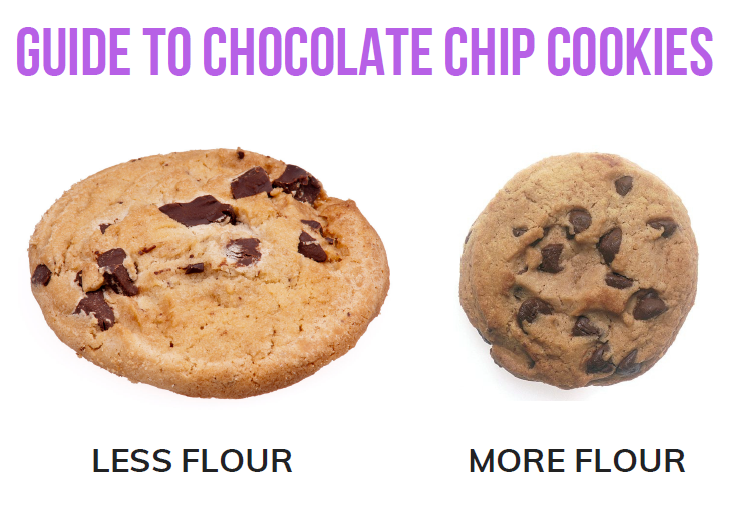# Fraction Operations

## Objective

Compare and order fractions greater than 1 using various methods.

## Common Core Standards

### Core Standards

?

• 4.NF.B.3.C — Add and subtract mixed numbers with like denominators, e.g., by replacing each mixed number with an equivalent fraction, and/or by using properties of operations and the relationship between addition and subtraction.

?

• 4.NF.A.1

• 4.NF.A.2

## Criteria for Success

?

1. Compare two fractions greater than 1 written as mixed numbers or as fractions by choosing the strategy that would be most efficient, such as comparing to a benchmark, finding a common numerator, or finding a common denominator.
2. Order a set of fractions greater than 1 written as mixed numbers or as fractions using various strategies by comparing two fractions in the set at a time.
3. Use the correct symbol (< , >, =) to record a comparison.

## Tips for Teachers

?

• Now that students have converted back and forth between fractions and mixed numbers, Lesson 11 returns to the comparison work students did in Unit 5 to compare and order fractions greater than 1 written in various forms. Because today’s lesson isn’t directly aligned to the unit’s focus of fraction operations, though, you may decide to cut this lesson and instead put similar tasks into previous and upcoming lessons to ensure they are able to generalize the work of Unit 5 to fractions greater than 1 without dedicating additional instructional time to it.
• Before the Problem Set, you could have students play a fraction comparison game "Making Fractions" described in the Georgia Standards of Excellence Curriculum Frameworks GSE Fourth Grade Unit 3: Fraction Equivalents on page 75. This game is similar to the one played in Unit 5.

#### Remote Learning Guidance

If you need to adapt or shorten this lesson for remote learning, we suggest prioritizing Anchor Task 3 (can be done independently). Find more guidance on adapting our math curriculum for remote learning here.

#### Fishtank Plus

• Problem Set
• Student Handout Editor
• Vocabulary Package

?

### Problem 1

Alex, Barbara, Caroline, and Damien need flour for their cookie recipes. They know that the amount of flour in a recipe will change what the cookies are like, according to the picture they found online:Alex’s recipes calls for ${{19\over8}}$ cups of flour, Barbara’s calls for ${2{3\over4}}$ cups of flour, Caroline’s calls for ${2{1\over3}}$ cups of flour, and Damien’s calls for ${{13\over4}}$ cups of flour. Which recipe calls for the most flour? Which recipe calls for the least? Show or explain your work.

#### References

Wikimedia Commons Photo: A Nantucket Pepperidge Farm Chocolate Chip Cookie by Evan-Amos

A Nantucket Pepperidge Farm Chocolate Chip Cookie is made available on Wikimedia Commons under the CC0 1.0 license. Accessed July 18, 2018, 11:02 a.m..

Wikimedia Commons Photo: Chocolate Chip Cookie by Renee Comet

Chocolate Chip Cookie is made available on Wikimedia Commons under the CC0 1.0 license. Accessed July 18, 2018, 11 a.m..

### Problem 2

Compare. Record your comparison with symbols <, >, or =.

a.   ${{19\over8}}$  _____   ${{11\over4}}$

b.   ${4{2\over5}}$  _____  ${4{6\over17}}$

c.   ${{16\over3}}$  _____  ${5{5\over15}}$

### Problem 3

Compare. Record your comparison with symbols <, >, or =.

a.   ${2{5\over8}}$  _____  ${2{1\over3}}$

b.   ${{13\over5}}$  _____  ${{20\over6}}$

c.   ${{35\over8}}$  _____  ${4{7\over12}}$

d.   ${6{1\over11}}$  _____  ${{41\over7}}$

## Problem Set & Homework

#### Discussion of Problem Set

• In what way is #1(c) easier than #1(d)?
• Who converted to a mixed number or a fraction greater than 1 before finding like units for #1(e)? Was it easier to compare mixed numbers or fractions greater than 1 for this particular problem?
• In #1(i), the denominators had no common factors. What strategy did you use to solve? Did you solve by finding like numerators or by drawing a model to find like denominators?
• At first glance, #3(j) looks really difficult. What makes it easier to solve?
• Were there any problems in #1 or #3 that you could compare without renaming or without drawing a model? How were you able to mentally compare them?
• When comparing the mixed numbers and fractions on the Problem Set, which strategies did you use? Were some strategies easier than others? Was it helpful to think about benchmark fractions?
• Why is it often easier to compare mixed numbers than to compare fractions greater than 1?
• How does this lesson relate to earlier lessons? How did earlier lessons help you to understand this lesson?

?

Compare the following fractions by writing >, <, or =.

1.   ${{22\over5}}$ _____ ${4{2\over7}}$

2.   ${3{1\over4}}$ _____ ${3{7\over12}}$

3.   ${5{7\over12}}$ _____ ${{23\over4}}$

4.   ${{29\over8}}$ _____ ${{12\over3}}$

### Mastery Response

?